Module 22 - Answers Lesson 1 Answer 1 22.1.1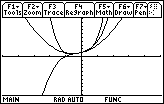The graphs appear to coincide approximately on the interval (-1/2, 1/2).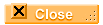Answer 2 22.1.2   The graph of y = 1/(1 - x) is in "thick" style and the graph of the tenth-degree partial sum y = 1 + x + x2 + ... + x10 =(xn, n, 0, 10) is in line style.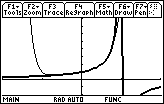Answer 3 22.1.3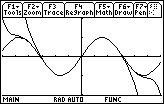The graphs appear to converge to y = sin x around x = 0.Answer 4 22.1.4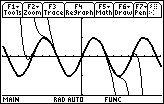Answer 5 22.1.5   The graphs are shown in [-10, 10] x [-10, 10] and [0, 10] x [0, 1000] viewing windows.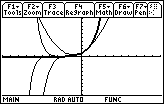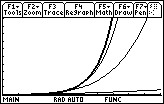As more terms are added, the interval where the partial sums are approximately the same as ex widens. The interval of convergence for the infinite power series is (–,)Lesson 2 Answer 1 22.2.1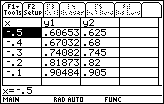The quadratic polynomial is 0.01847 greater than the actual value of y = ex when x = -0.5 and 0.02372 less than the actual value of y = ex when x = 0.5.Answer 2 22.2.2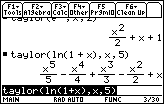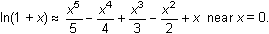Answer 3 22.2.3   The graphs of y = ln(1 + x) (thick) and the 5th-order polynomial (line) are shown in a [-7.9, 7.9] x [-3, 3] window.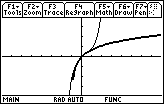Lesson 3 Answer 1 22.3.1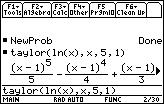Near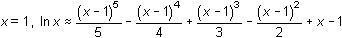.Answer 2 22.3.2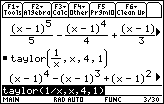Near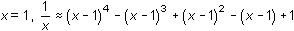.Answer 3 22.3.3  The derivative of the fifth-degree Taylor polynomial centered at 1 for y = ln x is equal to the fourth-degree Taylor polynomial centered at 1 for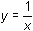. This seems reasonable because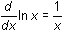.Answer 4 22.3.4 The derivative of the fifth- degree Maclaurin polynomial for y = sin x should be equal to the fourth- degree Maclaurin polynomial for y = cos x.Self Test Answer 1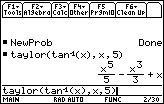Answer 2 The graphs are shown in a [-2, 2] x [-2, 2] window.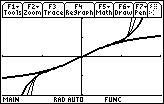The interval of convergence appears to be (-1,1). Answer 3Answer 4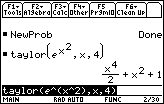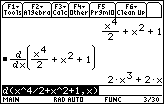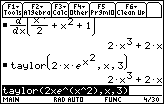Answer 5 The 5th-order Maclaurin polynomial for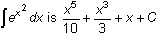©Copyright 2007 All rights reserved. | Trademarks | Privacy Policy | Link Policy